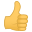# OtherModulus with magic number

#### freejlr

Hi guys.

I am reading a article that talks about how to calculate magic numbers to be able to optimize divisions, and in my case also the remainder.

This is my problem, I would like to be able to calculate the remainder in order to have the mod operator.

Well, create the magic numbers of 23 and 255 from the formula provided in the paper:

Code:
``````//DIV 23

movq \$10000, %rax
movq \$0x0b21642c8590b217,%rdx
mulq %rdx

//DIV 255

movq \$10000, %rax
movq \$0x101010101010102,%rdx
mulq %rdx``````

In the case of 255, when I operate the remainder remains in rax and the result in rdx like so:

Div 255:
rax= 0x3737373737375e20
rdi= 0x27

Well the result of rdx is correct since the division of 10,000 by 255 is 39.

In rax we can find the remainder (mod) in such a strange way that I don't know how to represent it, if we could do some bit movements, but what would be the correct formula?

10,000 mod 255 = 55 (0x37)

With 23 the result of the division is correct, but the result of the remainder is completely different from that of 255, I do not distinguish anything it would be equal to this:

Div 23:

rdx=0xc8590b21642ca270
rax=0x1b2

I disassembled some codes in C since Clang with the -O3 option generates magic numbers, but I didn't find anything clear, I couldn't solve the doubt, if it performs some arithmetic shift and shif, but I didn't understand the method.

Does anyone know the formula for how to obtain the mod for this type of division?

Regards.

P.S:

The article Magic number

#### Paul Floyd

"Hacker's Delight" 3ed has a section (10-3) on "Signed Division and Remainder by Non-Powers of 2". Your link has an acknowledgement to this source.

This starts by saying

"The basic trick is to multiply by a sort of reciprocal of the divisor d, approximately 2^32/d, and then to extract the leftmost 32 bits of the product"

•freejlr